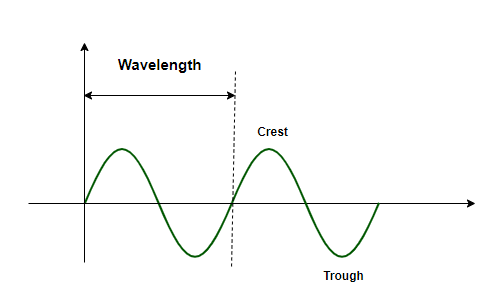GeeksforGeeks App
Open AppBrowser
Continue

# Light Waves And Color Formula

Light waves, like other kinds of waves, transport energy from a source. They are made up of discrete energy particles known as photons. Photons have no mass, yet they have momentum and energy. There are kinds of light waves that cannot be seen by human vision, just as there are sounds that cannot be heard because they are outside the range of human hearing. Wavelength and frequency, on the other hand, are shared by all forms of waves. The distance between adjacent portions of a wave is defined as its wavelength (e.g., crest to crest or trough to trough). The number of waves that pass at a fixed place at a particular time, on the other hand, is referred to as frequency.### Light Waves and Color Formula

Light waves may be measured in two ways.

• The wave’s amplitude.
• The frequency of the wave, as well as the number of waves each second.

Objects seem different colors because they absorb some colors (wavelength) while reflecting or transmitting others. The wavelengths that are reflected or transmitted by the item are what we experience as colors. So, based on the aforementioned premise, we may conclude that the color of light is determined by wavelength. Light wavelength and frequency are also strongly connected. The shorter the wavelength, the higher the frequency. Hence it is both- the wavelength as well as the frequency that determines the color of a light wave.

These waves are classified based on the frequencies that exhibit comparable properties in the electromagnetic spectrum of light. Each spectrum’s subdivisions differ depending on the wave frequency. The color of these lights is determined by the degree of reflection from the objects on which the white light falls. For example, the white wall appears because it reflects all of the colors back.

Formula

For electromagnetic waves, the following equation is used to relate wavelength and frequency:

c = vλ

Where,

• c depicts the speed of light
• v is the frequency
• λ depicts the wavelength

### Sample Problems

Question 1: If a wave has a frequency of 1.5 × 1014, find its wavelength.

Solution:

Given: v = 1.5 × 1014 and c = 3 × 108 m/s

Since, c = vλ ⇒ λ = c/v

⇒  λ = 3 × 108 m/s/1.5 × 1014

λ = 2 × 10-6 m.

Question 2: The wavelength of a light wave is made 10 times of its value. Explain what would happen to its frequency.

Clearly the wavelength and frequency of a light wave are inverse of each other. It implies that raising the wavelength by a certain factor would mean that the frequency of the said wave would reduce by the same factor.

Hence in this case, the frequency of the light wave would fall to one- tenth of its value.

Question 3: Calculate the frequency of red light, given that its frequency is 700 nm.

Solution:

So we are given that λ = 700 nm = 7.00 × 10-7 m.

Since, c = vλ ⇒ v = c/λ

⇒ v = 3 × 108 m/s/7 × 10-7 m

⇒ v = 0.4285 × 1015 ms-1

⇒ v = 4.285 × 1014 ms-1

Question 4: Find the color of a light wave of frequency 5.893 × 1014 s−1?

Solution:

Given: v = 5.893 ×1014s−1

Since, c = vλ ⇒ λ = c/v

⇒ λ = 3 × 108 m/s/5.893 × 1014s−1

⇒ λ = 5.0907 × 10-5 m

= 509 nm

Since the wavelength of green color lies between 490 – 530 nm, the color of the given wave is green.

Question 5: A ripple tank generates 10 ripples every second. Calculate the frequency, wavelength, and velocity of a wave if a trough and a nearby crest are 12 cm apart.

Solution:

The frequency of the wave is the same as the number of ripples per second.

Hence, frequency = v = 10 Hz.

Wavelength = λ = 2(0.12) m

= 0.24 m

Velocity = Frequency × wavelength

v × λ = 10 × 0.24

= 2.4 m/s

My Personal Notes arrow_drop_up
Related Tutorials